# 1. 引言

## 0x1：从维灾难说起

• “同质状”
• “环状”
• “薄片状”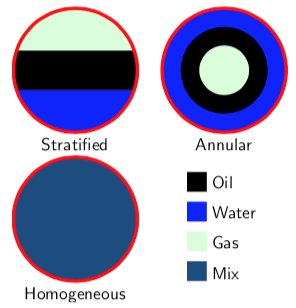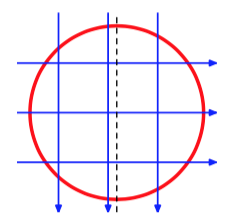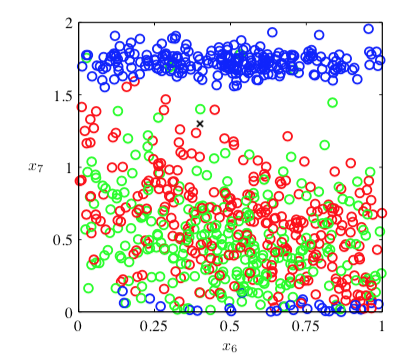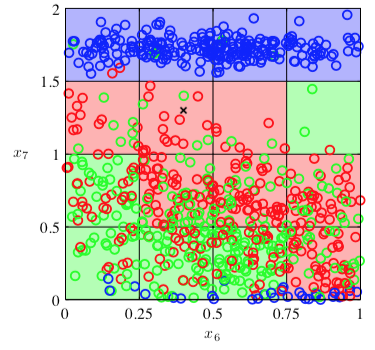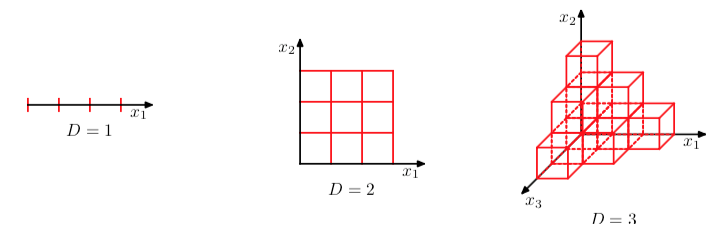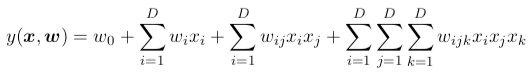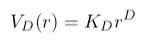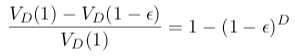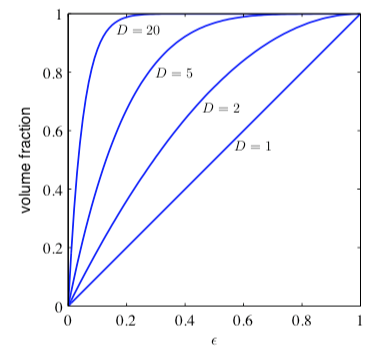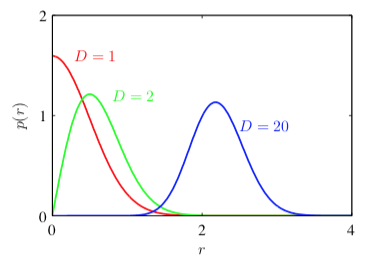• 第一，真实的数据经常被限制在有着较低的有效维度的空间区域中，特别地，在目标值会发生重要变化的方向上也会有这种限制。
• 第二，真实数据通常比较光滑(至少局部上比较光滑)，因此大多数情况下，对于输入变量的微小改变，目标值的改变也很小，因此对于新的输入变量，我们可以通过局部的类似于插值的技术来进行预测。

## 0x3：为什么要降维

1. 首先，高维数据增加了运算的难度
2. 其次，高维使得学习算法的泛化能力变弱（例如，在最近邻分类器中，样本复杂度随着维度成指数增长），维度越高，算法的搜索难度和成本就越大。
3. 最后，降维能够增加数据的可读性，利于发掘数据的有意义的结构

malware detection这种non-linear分类问题中，我们提取的feature往往是sparce high-dimension vector(稀疏高维向量)，典型地例如对malware binary的code .text section提取byte n-gram，这个时候，x轴(代码段的byte向量)高达45w，再乘上y轴(最少也是256)，直接就遇到了维数灾难问题，导致神经网络求解速度极慢，甚至内存MMO问题。

Relevant Link:

http://www.tomshardware.com/news/deep-instinct-deep-learning-malware-detection,31079.html
https://www.computerpoweruser.com/article/18961/israeli-company-aims-to-be-first-to-apply-deep-learning-to-cybersecurity
https://www.technologyreview.com/s/542971/antivirus-that-mimics-the-brain-could-catch-more-malware/
https://www.microsoft.com/en-us/research/wp-content/uploads/2016/02/MalwareRandomProjections.pdf
http://e-nns.org/
https://arxiv.org/pdf/1703.02244.pdf
http://www.dartmouth.edu/~gvc/
http://www.cs.toronto.edu/~gdahl/ 

# 2. PCA主成分分析(Principal components analysis)

## 0x1：PCA算法模型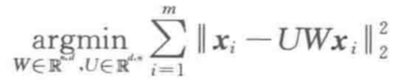，即尽量无损压缩。

PCA(Principal Component Analysis)是一种常用的数据分析方法。PCA通过线性变换将原始数据变换为一组各维度线性无关（单位正交）的表示，可用于提取数据的主要特征分量，常用于高维数据的降维。

## 0x2: 在讨论PCA约简前先要讨论向量的表示及基变换 - PCA低损压缩的理论基础

### 1. 内积与投影

(a1,a2,⋯,an)T⋅(b1,b2,⋯,bn)T=a1b1+a2b2+⋯+anbn### 2. 基### 3. 基变换的矩阵表示（R x N）. （N x M）=（R x M）

## 0x3: 协方差矩阵及优化目标 - 如何找到损失最低的变换基### 1. 投影后的新坐标点的方差 - 一种表征信息丢失程度的度量### 2. 协方差将一组N维向量降为K维(K大于0，小于N)，其目标是选择K个单位(模为1)正交基，使得原始数据变换到这组基上后，各字段两两间协方差为0(各自独立)；而字段的方差则尽可能大(投影后的点尽可能离散)。在正交的约束下，取最大的K个方差

### 3. 协方差矩阵 - 字段内方差及字段间协方差的统一数学表示### 4. 协方差矩阵对角化1）实对称矩阵不同特征值对应的特征向量必然正交。
2）设特征向量λλ重数为r，则必然存在r个线性无关的特征向量对应于λλ，因此可以将这r个特征向量单位正交化。P是协方差矩阵的特征向量单位化后按行排列出的矩阵，其中每一行都是C的一个特征向量。如果设P按照ΛΛ中特征值的从大到小，将特征向量从上到下排列，则用P的前K行组成的矩阵乘以原始数据矩阵X，就得到了我们需要的降维后的数据矩阵Y

## 0x4：PCA优化问题的解 - 和协方差矩阵对角化的关系，即尽量无损压缩。

1. 降维后的信息损失尽可能小，尽可能保留原始样本的概率分布
2. 降维后的基之间是完全正交的

## 0x5: PCA算法过程

### 1. PCA算法过程公式化描述

1. 设有m条n维数据

2. 将原始数据按列组成n行m列矩阵X

3. 将X的每一行(代表一个属性字段，即一个维度)进行零均值化，即减去这一行的均值

4. 求出协方差矩阵5. 求出协方差矩阵的特征值（矩阵特征值）及对应的特征向量（矩阵特征向量）

6. 将特征向量按对应特征值大小从上到下按行排列成矩阵，取前k行组成矩阵P

7.即为降维到k维后的数据。

### 2. 一个例子PCA也存在一些限制

## 0x7: 基于原生python+numpy实现PCA算法

1. 零均值化

def zeroMean(dataMat):
meanVal=np.mean(dataMat,axis=0)     #按列求均值，即求各个特征的均值
newData=dataMat-meanVal
return newData,meanVal  

2. 求协方差矩阵

newData,meanVal=zeroMean(dataMat)
covMat=np.cov(newData,rowvar=0)  

numpy中的cov函数用于求协方差矩阵，参数rowvar很重要！若rowvar=0，说明传入的数据一行代表一个样本，若非0，说明传入的数据一列代表一个样本。因为newData每一行代表一个样本，所以将rowvar设置为0

3. 求特征值、特征矩阵

eigVals,eigVects=np.linalg.eig(np.mat(covMat))

eigVals存放特征值，行向量。
eigVects存放特征向量，每一列带别一个特征向量。

4. 保留主要的成分[即保留值比较大的前n个特征]

eigValIndice=np.argsort(eigVals)            #对特征值从小到大排序
n_eigValIndice=eigValIndice[-1:-(n+1):-1]   #最大的n个特征值的下标
n_eigVect=eigVects[:,n_eigValIndice]        #最大的n个特征值对应的特征向量
lowDDataMat=newData*n_eigVect               #低维特征空间的数据
reconMat=(lowDDataMat*n_eigVect.T)+meanVal  #重构数据
return lowDDataMat,reconMat 

5. 完整code

# 零均值化
def zeroMean(dataMat):
meanVal = np.mean(dataMat, axis=0)  # 按列求均值，即求各个特征的均值
newData = dataMat - meanVal
return newData, meanVal

def pca(dataMat, n):
newData, meanVal = zeroMean(dataMat)
covMat = np.cov(newData, rowvar=0)  # 求协方差矩阵,return ndarray；若rowvar非0，一列代表一个样本，为0，一行代表一个样本

eigVals, eigVects = np.linalg.eig(np.mat(covMat))  # 求特征值和特征向量,特征向量是按列放的，即一列代表一个特征向量
eigValIndice = np.argsort(eigVals)  # 对特征值从小到大排序
n_eigValIndice = eigValIndice[-1:-(n + 1):-1]  # 最大的n个特征值的下标
n_eigVect = eigVects[:, n_eigValIndice]  # 最大的n个特征值对应的特征向量
lowDDataMat = newData * n_eigVect  # 低维特征空间的数据
reconMat = (lowDDataMat * n_eigVect.T) + meanVal  # 重构数据
return lowDDataMat, reconMat

Relevant Link:

http://www.cnblogs.com/jerrylead/archive/2011/04/18/2020209.html
http://blog.codinglabs.org/articles/pca-tutorial.html

## 0x8: 对图像数据应用PCA算法

1. 特征的均值大致为0
2. 不同特征的方差值彼此相似

### 1. 利用PCA进行人脸识别# -*- coding: utf-8 -*-

import numpy as np
import scipy.linalg as linA # 为了激活线性代数库mkl
from PIL import Image
from resizeimage import resizeimage
import os,glob

imageWidth = 230
imageHigth = 300
imageSize = imageWidth * imageHigth

def sim_distance(train,test):
'''
计算欧氏距离相似度
:param train: 二维训练集
:param test: 一维测试集
:return: 该测试集到每一个训练集的欧氏距离
'''
return [np.linalg.norm(i - test) for i in train]

def resizeImage(filepath):
img = Image.open(filepath)
img = img.resize((imageWidth, imageHigth), Image.BILINEAR)
img.save(filepath)

def resizeImages():
picture_path = os.getcwd() + '/images/'
for name in glob.glob(picture_path + '*.jpeg'):
print name
resizeImage(name)

def calcVector(arr1, arr2):
distance1, distance2 = 0, 0
for i in arr1:
distance1 += i * i
distance1 = distance1 / len(arr1)
for i in arr2:
distance2 += i * i
distance2 = distance2 / len(arr2)

return distance1 < distance2

def main():
picture_path = os.getcwd() + '/images/'
print "picture_path: ", picture_path
array_list = []
for name in glob.glob(picture_path + '*.jpeg'):
print name
# 读取每张图片并生成灰度（0-255）的一维序列 1*120000
img = Image.open(name)
# img_binary = img.convert('1') 二值化
img_grey = img.convert('L')  # 灰度化
array_list.append(np.array(img_grey).reshape((1, imageSize)))  # 拉长为1维

mat = np.vstack((array_list))  # 将上述多个一维序列(每个序列代表一张图片)合并成矩阵 3*69000
P = np.dot(mat, mat.transpose())  # 计算P
v, d = np.linalg.eig(P)  # 计算P的特征值和特征向量
print 'P Eigenvalues'
print v
print "Feature vector"
print d

d = np.dot(mat.transpose(), d)  # 计算Sigma的特征向量 69000 * 3
train = np.dot(d.transpose(), mat.transpose())  # 计算训练集的主成分值 3*3
print '训练集pca降维后的向量数组'
print train

# 开始测试
# 用于测试的图片也需要resize为和训练基准样本集相同的size
resizeImage('images/test_1.jpg')
test_pic = np.array(Image.open('images/test_1.jpg').convert('L')).reshape((1, imageSize))
# 计算测试集到每一个训练集的欧氏距离
result1 = sim_distance(train.transpose(), np.dot(test_pic, d))
print 'test_1.jpg 降维后的向量'
print result1

resizeImage('images/test_2.jpg')
test_pic = np.array(Image.open('images/test_2.jpg').convert('L')).reshape((1, imageSize))
result2 = sim_distance(train.transpose(), np.dot(test_pic, d))
print 'test_2.jpg 降维后的向量'
print result2

# 欧式距离最小的即为最接近训练样本集的测试样本
if calcVector(result1, result2):
print 'test_1.jpg is a human'
else:
print 'test_2.jpg is a human'

if __name__ == '__main__':
resizeImages()
main()

/System/Library/Frameworks/Python.framework/Versions/2.7/bin/python /Users/zhenghan/PycharmProjects/littlehann/just4fun.py
/Users/zhenghan/PycharmProjects/littlehann/images/train_2.jpeg
/Users/zhenghan/PycharmProjects/littlehann/images/train_3.jpeg
/Users/zhenghan/PycharmProjects/littlehann/images/train_1.jpeg
picture_path:  /Users/zhenghan/PycharmProjects/littlehann/images/
/Users/zhenghan/PycharmProjects/littlehann/images/train_2.jpeg
/Users/zhenghan/PycharmProjects/littlehann/images/train_3.jpeg
/Users/zhenghan/PycharmProjects/littlehann/images/train_1.jpeg
P Eigenvalues
[ 444.76007266 -199.2827456    -8.47732705]
Feature vector
[[-0.557454   -0.7252759   0.40400484]
[-0.69022539  0.1344664  -0.71099065]
[-0.46133931  0.67519898  0.57556266]]
pca
[[ -2.94130809e+09  -2.81400683e+09  -2.27967171e+09]
[ -4.53920521e+08   2.41231868e+07   4.49796574e+07]
[  5.06334430e+08   1.43429000e+08   2.56660545e+08]]
test_1.jpg
[859150941.34167683, 507130780.35877681, 98296821.771007225]
test_2.jpg
[921097812.32432926, 784122768.95719075, 323861431.46721846]
test_1.jpg is a human

Process finished with exit code 0test_1.jpg
[859150941.34167683, 507130780.35877681, 98296821.771007225]
test_2.jpg
[921097812.32432926, 784122768.95719075, 323861431.46721846]
test_1.jpg is a humansklearn.decomposition.PCA(n_components=None, copy=True, whiten=False)

1. n_components: PCA算法中所要保留的主成分个数n，也即保留下来的特征个数n
1) 缺省时默认为None，所有成分被保留
2) 赋值为int，比如n_components=1，将把原始数据降到一个维度
3) 赋值为string，比如n_components='mle'，将自动选取特征个数n，使得满足所要求的方差百分比。
2. copy: 表示是否在运行算法时，将原始训练数据复制一份。若为True，则运行PCA算法后，原始训练数据的值不会有任何改变，因为是在原始数据的副本上进行运算；若为False，则运行PCA算法后，原始训练数据的值会改，因为是在原始数据上进行降维计算
1) 缺省时默认为True
3. whiten: 白化，使得每个特征具有相同的方差(即去均值化)
1) 缺省时默认为False# -*- coding: utf-8 -*-

from sklearn.decomposition import PCA

data = [[ 1.  ,  1.  ],
[ 0.9 ,  0.95],
[ 1.01,  1.03],
[ 2.  ,  2.  ],
[ 2.03,  2.06],
[ 1.98,  1.89],
[ 3.  ,  3.  ],
[ 3.03,  3.05],
[ 2.89,  3.1 ],
[ 4.  ,  4.  ],
[ 4.06,  4.02],
[ 3.97,  4.01]]

if __name__ == '__main__':
pca = PCA(n_components=1)
newData = pca.fit_transform(data)
print newData

## 0x9: 选择主成分个数def percentage2n(eigVals,percentage):
sortArray=np.sort(eigVals)   #升序
sortArray=sortArray[-1::-1]  #逆转，即降序
arraySum=sum(sortArray)
tmpSum=0
num=0
for i in sortArray:
tmpSum+=i
num+=1
if tmpSum>=arraySum*percentage:
return num

def pca(dataMat,percentage=0.99):
newData,meanVal=zeroMean(dataMat)
covMat=np.cov(newData,rowvar=0)    #求协方差矩阵,return ndarray；若rowvar非0，一列代表一个样本，为0，一行代表一个样本
eigVals,eigVects=np.linalg.eig(np.mat(covMat))#求特征值和特征向量,特征向量是按列放的，即一列代表一个特征向量
n=percentage2n(eigVals,percentage)                 #要达到percent的方差百分比，需要前n个特征向量
eigValIndice=np.argsort(eigVals)            #对特征值从小到大排序
n_eigValIndice=eigValIndice[-1:-(n+1):-1]   #最大的n个特征值的下标
n_eigVect=eigVects[:,n_eigValIndice]        #最大的n个特征值对应的特征向量
lowDDataMat=newData*n_eigVect               #低维特征空间的数据
reconMat=(lowDDataMat*n_eigVect.T)+meanVal  #重构数据
return lowDDataMat,reconMat  

Relevant Link:

http://ufldl.stanford.edu/wiki/index.php/%E4%B8%BB%E6%88%90%E5%88%86%E5%88%86%E6%9E%90
http://blog.csdn.net/watkinsong/article/details/823476
http://www.cnblogs.com/theskulls/p/4925147.html
http://ufldl.stanford.edu/wiki/index.php/%E5%AE%9E%E7%8E%B0%E4%B8%BB%E6%88%90%E5%88%86%E5%88%86%E6%9E%90%E5%92%8C%E7%99%BD%E5%8C%96
http://book.2cto.com/201406/43853.html
http://pythoncentral.io/resize-image-python-batch/
https://opensource.com/life/15/2/resize-images-python
https://pypi.python.org/pypi/python-resize-image
https://docs.scipy.org/doc/numpy-1.10.0/reference/generated/numpy.ndarray.resize.html
http://www.ctolib.com/topics-58310.html
http://www.cnblogs.com/chenbjin/p/4200790.html
http://blog.csdn.net/u012162613/article/details/42177327
http://blog.csdn.net/u012162613/article/details/42192293

# 3. Random Projection（随机投影）

## 0x2: Johnson–Lindenstrauss lemma，随机投影有效性的理论依据

### 2. 问题证明

JL理论证明了解决这个问题的可能性，即这种映射理论上是存在的。
 Johnson-Lindenstrauss Theorem For any 0 < ε < 1 and any positive integer n, let k be a positive integer such that$k\ge4(\epsilon^2/2-\epsilon^3/3)^{-1}\ln n$Then for any set V of n points in$\mathbf{R}^d$, there is a map$f:\mathbf{R}^d\rightarrow\mathbf{R}^k$ such that for all$u,v\in V$,$(1-\epsilon)\|u-v\|^2\le\|f(u)-f(v)\|^2\le(1+\epsilon)\|u-v\|^2$.Furthermore, this map can be found in expected polynomial time.

## 0x3: Random projection算法

### 1. Gaussian random projection - 高斯随机投影

#### 1）考虑在二维情况下如何获得均匀采样？

1. 先在一个包含该圆形的外接正方形内均匀的采样
2. 第二，将采样到的点投影到圆形上
# 具体地说就是
1. 先独立均匀的从区间[−1,1](我们假设圆形跟正方形的中心点都在原点)内产生两个值组成一个二维的点(x1,x2)
2. 将该二维点投影到圆形上#### 2）利用高斯分布在高维球体表面产生均匀分布点的方法

# -*- coding: utf-8 -*-

import numpy as np
from sklearn import random_projection

if __name__ == '__main__':
X = np.random.rand(100, 10000)
transformer = random_projection.GaussianRandomProjection()
X_new = transformer.fit_transform(X)
print X_new.shape

output:
(100, 3947)

Relevant Link:

http://blog.csdn.net/ljj583905183/article/details/47980169
http://cjbme.csbme.org/CN/abstract/abstract500.shtm

### 2. a uniform random k-dimensional subspace.

 Let A be a random$k\times d$ matrix that projects$\mathbf{R}^d$ onto a uniform random k-dimensional subspace.Multiply A by a fixed scalar$\sqrt{\frac{d}{k}}$. For every$v\in\mathbf{R}^d$, v is mapped to$\sqrt{\frac{d}{k}}Av$.

构造后的点$\sqrt{\frac{d}{k}}Av$ 是$\mathbf{R}^k$的其中一个向量.

### 3. Sparse random projection

# -*- coding: utf-8 -*-

import numpy as np
from sklearn import random_projection

if __name__ == '__main__':
X = np.random.rand(100, 10000)
transformer = random_projection.SparseRandomProjection()
X_new = transformer.fit_transform(X)
print X_new.shape

output:
(100, 3947)

### 4. More computationally efficient random projections

the Gaussian distribution can be replaced by a much simpler distribution such as## 0x4: Random projection在手写图像识别里的具体应用

### 1. 计算100%完全分类的baseline基线值

# -*- coding: utf-8 -*-

from sklearn.random_projection import johnson_lindenstrauss_min_dim

if __name__ == '__main__':
print johnson_lindenstrauss_min_dim(1797,eps=0.1)

Out:
6423

>>> from sklearn.random_projection import johnson_lindenstrauss_min_dim
>>> johnson_lindenstrauss_min_dim(n_samples=1e6, eps=0.5)
663
>>> johnson_lindenstrauss_min_dim(n_samples=1e6, eps=[0.5, 0.1, 0.01])
array([    663,   11841, 1112658])
>>> johnson_lindenstrauss_min_dim(n_samples=[1e4, 1e5, 1e6], eps=0.1)
array([ 7894,  9868, 11841])### 2. random projection的components个数是否和欧几里德分类准确度是正相关的

scikit_learn给出的baseline是6423，这显然太大了，我们的目的是降维，是为了提高运算效率的，为此，我们选取的components一定是小于64(原始8*8的图像维度)的。

# -*- coding: utf-8 -*-

from sklearn.random_projection import SparseRandomProjection
from sklearn.svm import LinearSVC
from sklearn.cross_validation import train_test_split
from sklearn import metrics
from sklearn import datasets
import matplotlib.pyplot as plt
import numpy as np

if __name__ == '__main__':
#  initializes our lists of accuracies. We’ll be evaluating 20 different component sizes, equally spaced from 2 to 64.
accuracies = []
components = np.int32(np.linspace(2, 64, 20))
print "components"
print components
'''
components
[ 2  5  8 11 15 18 21 24 28 31 34 37 41 44 47 50 54 57 60 64]
'''

# load the digits data set, perform a training and testing split, train a Linear SVM, and obtain a baseline accuracy:
digits = datasets.load_digits()
split = train_test_split(digits.data, digits.target, test_size=0.3, random_state=42)
(trainData, testData, trainTarget, testTarget) = split

model = LinearSVC()
model.fit(trainData, trainTarget)
baseline = metrics.accuracy_score(model.predict(testData), testTarget)

# loop over the projection sizes
'''
1. We start looping over our number of components, equally spaced, in the range 2 to 64.
2. Then we instantiate our SparseRandomProjection using the current number of components, fit our random projection to the data (which essentially means generating a sparse matrix of values), and then transforming our original training data by projecting the data.
3. Now that we have obtained a sparse representation of the data, let’s train a Linear SVM on it.
4. Our Linear SVM is now trained; it’s time to see how it performs on the testing data and update our list of accuracies.
'''
for comp in components:
# create the random projection
sp = SparseRandomProjection(n_components=comp)
X = sp.fit_transform(trainData)

# train a classifier on the sparse random projection
model = LinearSVC()
model.fit(X, trainTarget)

# evaluate the model and update the list of accuracies
test = sp.transform(testData)
accuracies.append(metrics.accuracy_score(model.predict(test), testTarget))

'''
At this point all the hard work is done.
We’ve evaluated a series of sparse random projections for varying numbers of components.
Let’s plot our results and see what we have:
'''
# create the figure
plt.figure()
plt.suptitle("Accuracy of Sparse Projection on Digits")
plt.xlabel("# of Components")
plt.ylabel("Accuracy")
plt.xlim([2, 64])
plt.ylim([0, 1.0])

# plot the baseline and random projection accuracies
plt.plot(components, [baseline] * len(accuracies), color="r")
plt.plot(components, accuracies)

plt.show()Relevant Link:

http://forum.ai100.com.cn/blog/thread/py-2015-02-19-3811867870631018/
https://en.wikipedia.org/wiki/Johnson%E2%80%93Lindenstrauss_lemma
https://en.wikipedia.org/wiki/Random_projection
http://blog.yhat.com/posts/sparse-random-projections.html
http://scikit-learn.org/stable/auto_examples/datasets/plot_digits_last_image.html
http://scikit-learn.org/stable/modules/random_projection.htmlhttps://blog.csdn.net/luoyun614/article/details/39853259

# 4. 压缩感知

https://www.cnblogs.com/Eufisky/p/7798767.html#commentform
https://blog.csdn.net/yq_forever/article/details/55271952
https://www.cnblogs.com/AndyJee/p/4988623.html

# 5. 主成分回归（principle component regression，PCR）

## 0x1：主成份的组成

PCA的主成分是通过选择原始属性在新的正交基上的投影向量的前k个来实现，和主成分分析PCA不同的是，PCR的主成分是基于原始属性的线性组合

Relevant Link:

https://www.jianshu.com/p/d090721cf501?from=timeline

# 5. 偏最小二乘回归（partial least-squares regression）

## 0x1：偏最小二乘计算方法

• 量纲归一化：从输入属性开始，所有属性被标准化为拥有零均值和单位方差
• 初始偏差计算：用于第一个偏最小二乘方向的属性系数是通过每一个属性向量和类向量之间以此进行点积运算得到的，点积结果代表方向上的偏差。
• 残差驱动的启发式属性选择：用同样的方法找到第二个方向，但是，此时的原始属性要被替换为，该属性原始值与用上一轮迭代选定的单变量属性回归所得的预测值之间的差值，这个单变量属性回归使用的是上一轮选定的属性（上一轮的残差）作为属性预测的单一预测因子。这些差值被称为残差（redidual）。每次的残差（新属性）都代表针对当残差的一个方向上的修正，然后再通过点积度量依然存在的方向上的偏差
• 用同样的方式继续运行此流程以得到其余的方向，用前一次迭代所得的残差作为属性形成输入来找到当前偏最小二乘的方向。
• PLSR结束：整个流程结束后得到的所有属性，就是不断修正方向以靠近目标类方向的属性集合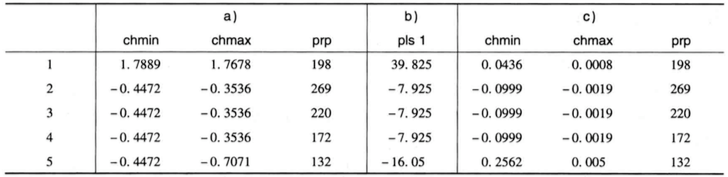CPU性能数据中的前5个实例

• 第一个偏最小二乘方向的属性系数是通过在属性和类属性之间依次进行点积运算得到的，表a）列出了原始属性值
• prp和chmin之间的点积是-0.4472
• prp和chmax之间的点积是22.981
• 因此，第一个偏最小二乘方向为：pls1 = -0.4472*chmin + 22.981*chmax，表b）列出了第一个偏最小二乘方向
• 接下来的步骤是准备输入数据，用以找到第二个偏最小二乘方向。为此，pls1依次回归到chmin和chmax，由pls1得到线性方程，用以单独预测这些属性中的每一个属性。这些系数通过计算pls1与待求解属性之间的点积得到，且用pls1与它自身的点积来划分所得的结果。由此产生的单变量（一元）回归方程为：
• chmin = 0.0483 * pls1
• chmax = 0.0444 * pls1
• 表c）列出的是准备用于寻找第二个偏最小二乘方向的CPU数据。chmin和chmax的原始值被残差所替代。残差是指原始值与之前给出的相应的单变量（一元）回归方程的输入之间的差值（目标值prp仍然一样，只是属性系数变了）。整个过程重复地使用这些数据作为输入产生第二个偏最小二乘方向，即：
• pls2 = -23.6002*chmin + -0.4593*chmax
• 在最后的偏最小二乘方向确定之后，属性的残差都为0。这反应了一个事实，正如主成分分析一样，所有方向的全集担负了原始数据的所有方差

• 将每个原始属性都看做是一个独立的神经元
• 每个神经元都是自己的独立的系数
• 所有神经元共同决定了最终的输出，同样，最终预测结果与目标值的差距，这个“错误责任”也要分担给所有的神经元
• 每个神经元都根据自己系数情况，根据一元回归的方式来度量自己的“偏离度”，并根据这个偏离度来进行对应的修改
• 经过不断地迭代，最终所有神经元都彼此协调，整体得到了一个适配目标值的局部最优结果

Relevant Link:

https://blog.csdn.net/qq_19600291/article/details/83823994
https://www.cnblogs.com/duye/p/9031511.html

# 7. 降维在其他领域的应用

## 0x1: PHP SSDEEP模糊化HASH

模糊化HASH也叫"局部不敏感HASH"，如果用于对比的两个数据集之间的区别只在一个很小的区域内，则模糊化HASH会忽略这种差别，的趋向于得到一个相等的Fuzzy HASH值

LSH算法的基本思想是利用一个hash函数把集合中的元素映射成hash值，使得相似度越高的元素hash值相等的概率也越高。LSH算法使用的关键是针对某一种相似度计算方法，找到一个具有以上描述特性的hash函数。LSH所要求的hash函数的准确数学定义比较复杂，以下给出一种通俗的定义方式：

对于集合S，集合内元素间相似度的计算公式为sim(*,*)。如果存在一个hash函数h(*)满足以下条件：存在一个相似度s到概率p的单调递增映射关系，使得S中的任意两个满足sim(a,b)>=s的元素a和b，h(a)=h(b)的概率大于等于p。那么h(*)就是该集合的一个LSH算法hash函数

Relevant Link:

http://www.cnblogs.com/GarfieldEr007/p/5479401.html

posted @ 2017-03-21 15:15  郑瀚Andrew.Hann  阅读(11184)  评论(5编辑  收藏  举报